# Vector Calculations

A LevelAQAEdexcelOCR

## Vector Calculations

Being able to use vectors in calculations is a key skill in A-Level, this section will cover the following areas of vector calculations:

• Finding vector magnitudes using Pythagoras’ theorem
• Resolving vectors into component form
• Finding the distance between points using a vector’s magnitude
• Using the cosine rule to find the angle between two vectors

Make sure you are happy with the following topics before continuing.

A LevelAQAEdexcelOCR

## Finding Vector Magnitudes Using Pythagoras’ Theorem

Notation: The magnitude of vector $\boldsymbol{a}$ is written as $|\boldsymbol{a}|$, similarly the magnitude of $\overrightarrow{AB}$ is written as $|\overrightarrow{AB}|$.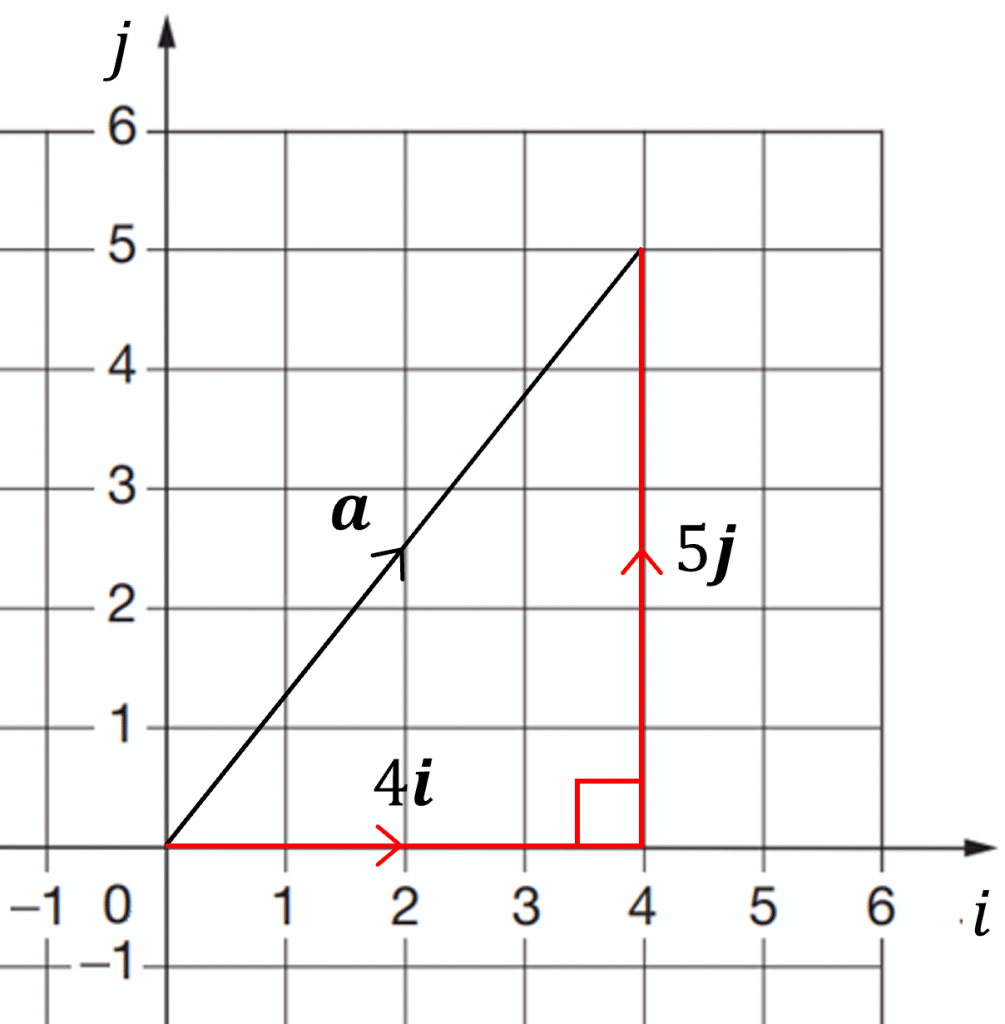As shown on the image on the right, the $\boldsymbol{i}$ and $\boldsymbol{j}$ components of a vector create a right-angled triangle, this means you can use Pythagoras’ theorem to find the magnitude (length) of the vector.

Using Pythagoras’ theorem we can calculate the magnitude of vector $\boldsymbol{a}$:

$|\boldsymbol{a}|=\sqrt{4^2+5^2}=\sqrt{41}$

Sometimes you may be required to find a unit vector in the direction of a particular vector, which can be found using:

Unit vector in the direction of a vector $\boldsymbol{a}=\dfrac{\boldsymbol{a}}{|\boldsymbol{a}|}$

As stated in position vectors, a unit vector has a magnitude of $1$

Example: Find the unit vector in the direction of $\boldsymbol{m}=-3\boldsymbol{i}+6\boldsymbol{j}$

We first need to find the magnitude of $\boldsymbol{m}$:

$|\boldsymbol{m}|=\sqrt{(-3)^2+6^2}=\sqrt{9+36}=3\sqrt{5}$

Therefore, the unit vector in the direction of $\boldsymbol{m}$ is:

$\dfrac{\boldsymbol{m}}{|\boldsymbol{m}|}=\dfrac{\boldsymbol{m}}{3\sqrt{5}}=\dfrac{1}{3\sqrt{5}}(-3\boldsymbol{i}+6\boldsymbol{j})$

A LevelAQAEdexcelOCR

## Resolving Vectors into Component Form

When calculating with vectors it is easier to work with them when they are separated into $\boldsymbol{i}$ and $\boldsymbol{j}$ vectors, allowing you to work with one component at a time.

Splitting a vector into components is called resolving the vector, which can be done using both trigonometry and Pythagoras’ theorem.

Example: An object is travelling at $11\text{ ms}^{-1}$ at an angle of $38\degree$ to the horizontal.

Find the horizontal $(\boldsymbol{i})$ and vertical $(\boldsymbol{j})$ components of the object’s velocity, $\boldsymbol{v}$.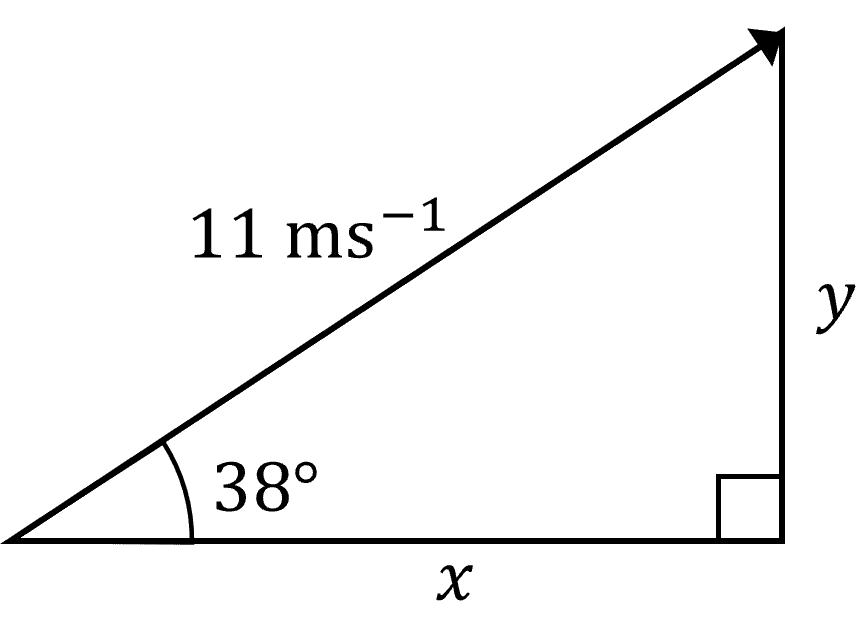It is often easier to draw a diagram and make a right angled triangle for the problem:

We can then use trigonometry to find $x$ and $y$:

$\cos{38\degree}=\dfrac{x}{11} \rArr x=11\cos{38\degree}$

$\sin{38\degree}=\dfrac{y}{11} \rArr y=11\sin{38\degree}$

Thus,

\begin{aligned} \boldsymbol{v}&=(11\cos{38\degree}\boldsymbol{i}+11\sin{38\degree}\boldsymbol{j})\text{ ms}^{-1} \\&=(8.67\boldsymbol{i}+6.77\boldsymbol{j})\text{ ms}^{-1} (2\text{ dp}) \end{aligned}

A LevelAQAEdexcelOCR

## Finding the Distance Between Points Using a Vector’s Magnitude

Calculating the distance between two points is fairly straight forward, you need to find the vector between them and then calculate its magnitude using Pythagoras’ theorem.

Example: The position vectors of points $K$ and $L$ are $6\boldsymbol{i}-2\boldsymbol{j}$ and $-3\boldsymbol{i}+5\boldsymbol{j}$ respectively.

Find the distance between the points $K$ and $L$, giving your answer to $2$ decimal places.

First, we need to find the vector $\overrightarrow{KL}$

$\overrightarrow{KL}=-3\boldsymbol{i}+5\boldsymbol{j}-(6\boldsymbol{i}-2\boldsymbol{j})=-9\boldsymbol{i}+7\boldsymbol{j}$

Then calculate the magnitude of $\overrightarrow{KL}$:

$|\overrightarrow{KL}|=\sqrt{(-9)^2+7^2}=\sqrt{130}=11.40 \, (2\text{ dp})$

A LevelAQAEdexcelOCR

## Using the Cosine Rule to Find the Angle Between Two Vectors

To find the angle between two vectors, $\boldsymbol{a}$ and $\boldsymbol{b}$, you can construct a triangle with $\boldsymbol{a}$ and $\boldsymbol{b}$ as two of the sides and then calculate the magnitude of $\overrightarrow{AB}$ for the remaining side. You can use the cosine rule to find the angle between the two vectors.

Example: $\overrightarrow{OA}=4\boldsymbol{i}+\boldsymbol{j}$ and $\overrightarrow{OB}=-2\boldsymbol{i}+5\boldsymbol{j}$

Find the angle $\theta$ between the vectors $\overrightarrow{OA}$ and $\overrightarrow{OB}$, giving your answer to $2$ decimal places.

We first need to calculate the magnitudes of the vectors, $\overrightarrow{OA},\overrightarrow{OB}$ and $\overrightarrow{AB}$, so we can construct a triangle.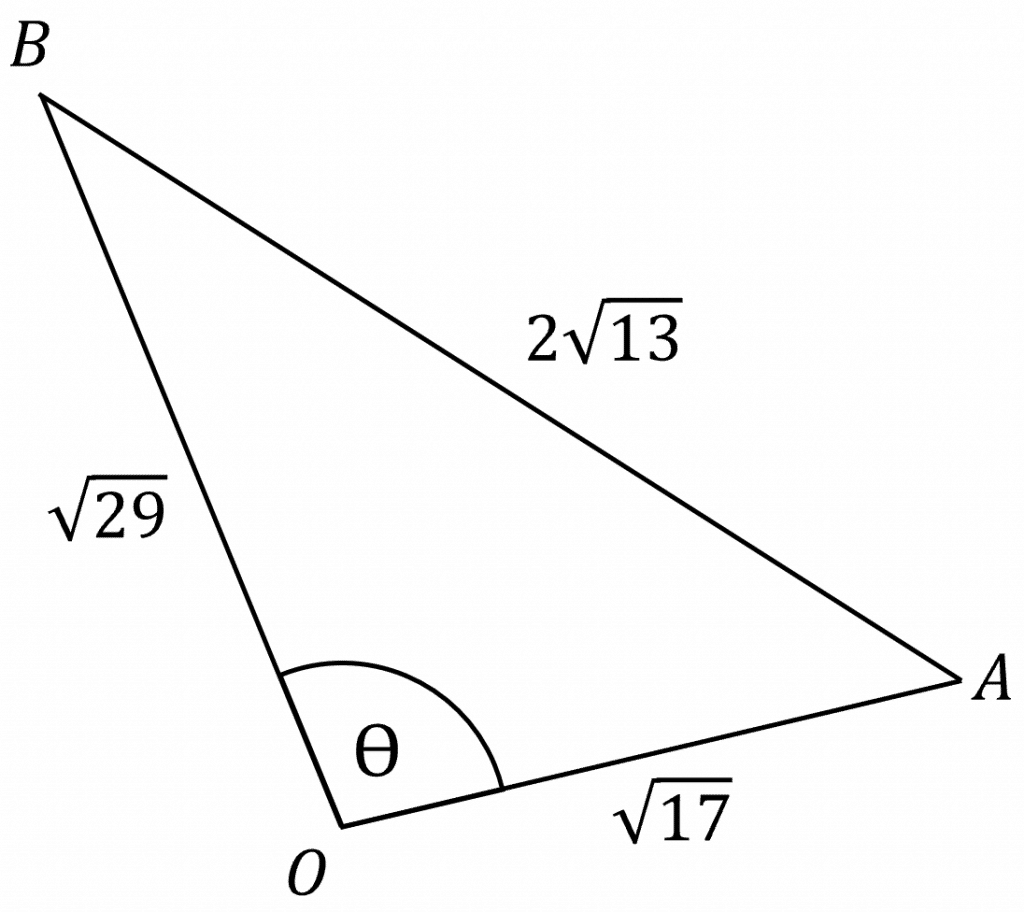$|\overrightarrow{OA}|=\sqrt{4^2+1^2}=\sqrt{17}$

$|\overrightarrow{OB}|=\sqrt{(-2)^2+5^2}=\sqrt{29}$

\begin{aligned} |\overrightarrow{AB}| &=|\overrightarrow{OB}-\overrightarrow{OA}| \\ &=|-2\boldsymbol{i}+5\boldsymbol{j}-(4\boldsymbol{i}+\boldsymbol{j})|\\ &=|-6\boldsymbol{i}+4\boldsymbol{j}| \\ &=\sqrt{(-6)^2+4^2}=2\sqrt{13} \end{aligned}

Then using the cosine rule to find angle $\theta$:

$\cos{\theta}=\dfrac{(\sqrt{17})^2+(\sqrt{29})^2-(2\sqrt{13})^2}{2\times \sqrt{17} \times \sqrt{29}}=\dfrac{-3}{\sqrt{493}}$

$\rArr \theta=\cos ^{-1} \left(\dfrac{-3}{\sqrt{493}} \right)=97.77 \degree \, (2\text{ dp})$

A LevelAQAEdexcelOCR

## Vector Calculations Example Questions

First find the magnitude of $\boldsymbol{t}$:

$|\boldsymbol{t}|=\sqrt{4^2+(-5)^2}=\sqrt{16+25}=\sqrt{41}$

Therefore, the unit vector is:

$\dfrac{\boldsymbol{t}}{|\boldsymbol{t}|}=\dfrac{\boldsymbol{t}}{\sqrt{41}}=\dfrac{1}{\sqrt{41}}(4\boldsymbol{i}-5\boldsymbol{j})$

First, we need to find the vector $\overrightarrow{ST}$ between the points.

$\overrightarrow{ST}=\dbinom{-5}{2}-\dbinom{4}{-3}=\dbinom{-5-4}{2-(-3)}=\dbinom{-9}{5}$

So, $|\overrightarrow{ST}|=\sqrt{(-9)^2+5^2}=\sqrt{106}=10.30 \, (2 \text{ dp})$

It is first useful to draw a diagram of the vector $\boldsymbol{w}$, forming a right-angled triangle.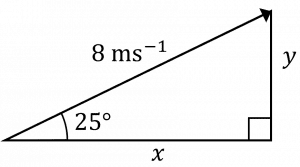Now we can use trigonometry to find $x$ and $y$:

$\cos{25\degree}=\dfrac{x}{8} \rArr x=8\cos{25\degree}$

$\sin{25\degree}=\dfrac{y}{8} \rArr y=8\sin{25\degree}$

So,

\begin{aligned} \boldsymbol{w}&=8\cos{25\degree}\boldsymbol{i}+8\sin{25\degree}\boldsymbol{j}\\ &=7.25\boldsymbol{i}+3.38\boldsymbol{j}\end{aligned}

We first need to find the following vectors: $\overrightarrow{XY}$, $\overrightarrow{YZ}$ and $\overrightarrow{XZ}$:

$\overrightarrow{XY}=-\boldsymbol{i}+2\boldsymbol{j}-(2\boldsymbol{i}+3\boldsymbol{j})=-3\boldsymbol{i}-\boldsymbol{j}$

$\overrightarrow{YZ}=4\boldsymbol{i}-2\boldsymbol{j}-(-\boldsymbol{i}+2\boldsymbol{j})=5\boldsymbol{i}-4\boldsymbol{j}$

$\overrightarrow{XZ}=4\boldsymbol{i}-2\boldsymbol{j}-(2\boldsymbol{i}+3\boldsymbol{j})=2\boldsymbol{i}-5\boldsymbol{j}$

Then, we need to find the magnitudes of those vectors so we can form a triangle:

$|\overrightarrow{XY}|=\sqrt{(-3)^2-(-1)^2}=\sqrt{10}$

$|\overrightarrow{YZ}|=\sqrt{5^2+(-4)^2}=\sqrt{41}$

$|\overrightarrow{XZ}|=\sqrt{2^2-(-5)^2}=\sqrt{29}$

This allows us to draw a triangle: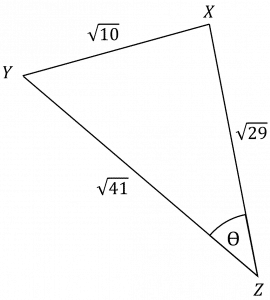Finally, using the cosine rule we can calculate the angle $\theta$:

$\cos{\theta}=\dfrac{(\sqrt{29})^2+(\sqrt{41})^2-(\sqrt{10})^2}{2\times\sqrt{29}\times \sqrt{41}}=\dfrac{60}{2\sqrt{1189}}=\dfrac{30}{\sqrt{1189}}$

$\rArr \theta=\cos^{-1}(\dfrac{30}{\sqrt{1189}})=29.54\degree \, (2\text{ dp})$

A Level

A Level

## You May Also Like...### MME Learning Portal

Online exams, practice questions and revision videos for every GCSE level 9-1 topic! No fees, no trial period, just totally free access to the UK’s best GCSE maths revision platform.

£0.00

A Level

A Level